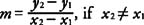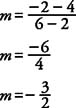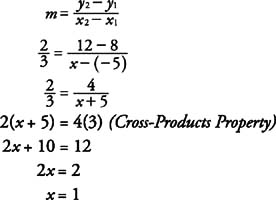## Slope of a Line

The slope of a line is a measurement of the steepness and direction of a nonvertical line. When a line rises from left to right, the slope is a positive number. Figure 1(a) shows a line with a positive slope. When a line falls from left to right, the slope is a negative number. Figure 1(b) shows a line with a negative slope. The x‐axis or any line parallel to the x‐axis has a slope of zero. Figure 1(c) shows a line whose slope is zero. The y‐axis or any line parallel to the y‐axis has no defined slope. Figure 1(d) shows a line with an undefined slope.Figure 1 Different possibilities for slope of a line.

If m represents the slope of a line and A and B are points with coordinates ( x ly 1) and ( x 2y 2) respectively, then the slope of the line passing through A and B is given by the following formula.A and B cannot be points on a vertical line, so x 1 and x 2 cannot be equal to one another. lf x 1 = x 2, then the line is vertical and the slope is undefined.

Example 1: Use Figure to find the slopes of lines a, b, c, and d.Figure 2 Finding the slopes of specific lines.

a. (a) Line a passes through the points (−7, 2) and (−3, 4).b. (b) Line b passes through the points (2, 4) and (6, −2).c. (c) Line c is parallel to the x‐axis. Therefore, m = 0.

d. (d) Line d is parallel to the y‐axis. Therefore, line d has an undefined slope.

Example 2: A line passes through (−5, 8) with a slope of 2/3. If another point on this line has coordinates ( x, 12), find x.